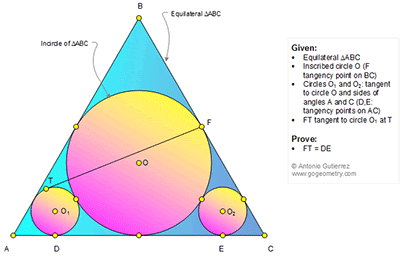## Sunday, February 19, 2017

### Geometry Problem 1314: Equilateral Triangle, Incircle, Inscribed Circle, Tangent, Congruence

Geometry Problem. Post your solution in the comment box below.
Level: Mathematics Education, High School, Honors Geometry, College.

Details: Click on the figure below.1.Problem 1314
Suppose the circle with center O intersects the circle O1 at point L. Bring the common tangent at the point L which intersects AB and AC in P, Q respectively.Draw OK perpendicular in AC (K on AC). The points A,L,O and F are collinear.Is <OAK=30
so OA=2OK=2r, and triangle APQ is equilateral, then AO1=2AL/3.But LO=KO, <LOK=60
then triangle LKO is equilateral so <LKA=0-60=30=<KAL.Therefore AL=LK=LO=OK=r.
So AO1=AO/3 and r1=O1D=OK/3=r/3. In triangle OTF ( <OTF=90) we have
FT^2=O1F^2-O1T^2=(2r+r1)^2-r1^2=4r(r+r1)=16r^2/3 (1).Draw O1M//DE (M on OK).
Is <O1MO=90 then O1M^2=OO1^2-OM^2=(r+r1)^2-(r-r1)^2=4rr1=4r^2/3. Now
DE^2=4DK^2=4O1M^2=16r^2/3 (2).Therefore FT=DE.
APOSTOLIS MANOLOUDIS KORYDALLOS PIRAEUS GREECE

2.Join F to A , T to O1, O to M ( midpoint AC)From Right tr with angle 30°
=> R = 3r => TF = 4r√3. From AOM => AC = 6r√3, AD=EC=r√3 => DE = 4r√3

3.Let the radius of O = r and that of O1 and O2 = u.

Since < O1AD = 30, r+u = 2(r-u)
hence r = 3u......(1)

DE^2 = 4{(r+u)^2 - (r-u)^2} = 48u^2 ..(2) from (1)

FT^2 = (2r+u)^2 - u^2 = 48u^2 ..(3) also from (1)

From (2) and (3) DE = FT

Sumith Peiris
Moratuwa
Sri Lanka